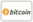Bookcover of Chromatic polynomials and chromaticity of some linear h-hypergraphs
Booktitle:

# Chromatic polynomials and chromaticity of some linear h-hypergraphs

VDM Verlag Dr. Müller (2011-04-08 )eligible for voucher
ISBN-13:

### 978-3-639-34823-1

ISBN-10:
3639348230
EAN:
9783639348231
Book language:
English
Blurb/Shorttext:
For a century, one of the most famous problems in mathematics was to prove the four-color theorem.In 1912, George Birkhoff proposed a way to tackling the four-color conjecture by introduce a function P(M, t), defined for all positive integer t, to be the number of proper t-colorings of a map M. This function P(M, t)in fact a polynomial in t is called chromatic polynomial of M. If one could prove that P(M, 4)>0 for all maps M, then this would give a positive answer to the four-color problem. In this book, we have proved the following results: (1)Recursive form of the chromatic polynomials of hypertree, Centipede hypergraph, elementary cycle, Sunlet hypergraph, Pan hypergraph, Duth Windmill hypergraph, Multibridge hypergraph, Generalized Hyper-Fan, Hyper-Fan, Generalized Hyper-Ladder and Hyper-Ladder and also prove that these hypergraphs are not chromatically uniquein the class of sperenian hypergraphs. (2)Tree form and Null graph representation of the chromatic polynomials of elementary cycle, uni-cyclic hypergraph and sunflower hypergrpah. (3)Generalization of a result proved by Read for graphs to hypergraphs and prove that these kinds of hypergraphs are not chromatically unique.
Publishing house:
VDM Verlag Dr. Müller
Website:
http://www.vdm-verlag.de
By (author) :
Number of pages:
120
Published at:
2011-04-08
Stock:
Available
Category:
Arithmetic, Algebra
Price:
49.00 €
Keywords:
" Hypergraphs, h-uniform hyper cycle, linear h-uniform path Chromatic Polynomial and Chromatic uniqueness"### Categories